# Past Seminar and Recordings

#### March 29, 2023:

###### Abstract:
In this talk, motivated by the calibration problem in local stochastic volatility models, we will investigate some McKean–Vlasov equations beyond the usual requirement of continuity of the coefficients in the measure variable for the Wasserstein topology. We will provide first an existence result for this type of McKean–Vlasov equations and explain the main idea behind the proof. In a second time, we will show an approximation by particle system for this type of equations, a result almost never rigorously proven in the literature in this context.

###### Abstract:

We propose a new method for solving optimal stopping problems (such as American option pricing in finance) under minimal assumptions on the underlying stochastic process X.
We consider classic and randomized stopping times represented by linear and non-linear functionals of the rough path signature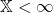associated to X, and prove that maximizing over these classes of signature stopping times, in fact, solves the original optimal stopping problem. Using the algebraic properties of the signature, we can then recast the problem as a(deterministic) optimization problem depending only on the (truncated) expected signature. By applying a deep neural network approach to approximate the non-linear signature functionals, we can efficiently solve the optimal stopping problem numerically.

The only assumption on the process X is that it is a continuous (geometric) random rough path. Hence, the theory encompasses processes such as fractional Brownian motion, which fail to be either semi-martingales or Markov processes, and can be used, in particular, for American-type option pricing in fractional models, e.g. of financial or electricity markets. (Based on joint work with Paul Hager, Sebastian Riedel, and John Schoenmakers)

#### March 8, 2023:

###### Abstract:

The Landau-Lifschitz-Gilbert equation is a model describing the magnetisation of a ferromagnetic material. The stochastic model is studied to observe the role of thermal fluctuations. We interpret the linear multiplicative noise appearing by means of rough paths theory and we study existence and uniqueness of the solution to the equation on a one dimensional domain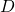. As a consequence of the rough paths formulation, the map that to the noise associates the unique solution to the equation is locally Lipschitz continuous in the strong norm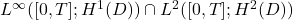. This implies a Wong-Zakai convergence result, a large deviation principle, a support theorem.

We prove also continuity with respect to the initial datum initial datum in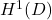, which allows to conclude the Feller property for the associated semigroup. We also discuss briefly a Path-wise central limit theorem and a moderate deviations principle for the stochastic LLG: in this case the rough paths formulation leads to a path-wise convergence, not easily reachable in the classical It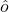‘s calculus setting.

The talk is based on a joint work with A. Hocquet [https://arxiv.org/abs/2103.00926] and on [https://arxiv.org/abs/2208.02136].

#### February 15, 2023:

###### Abstract:

In this talk, I will present several results on the Anderson Hamiltonian with white noise potential in dimension 1. This operator formally writes « – Laplacian + white noise ». It arises as the scaling limit of various discrete models and its explicit potential allows for a detailed description of its spectrum. We will discuss localization of its eigenfunctions as well as the behavior of the local statistics of its eigenvalues. Around large energies, we will see that the eigenfunctions are delocalized and follow a universal shape given by the exponential of a Brownian motion plus a drift, a behavior already observed by Rifkind and Virag in tridiagonal matrix models. Based on joint works with Cyril Labbé.

#### February 8, 2023:

###### Title: On conditional densities of partially observed jump diffusions

Abstract: I will present the filtering problem for a partially observed jump diffusion system Z=(X,Y) driven by Wiener processes and Poisson martingale measures. We will derive the filtering equations, describing the time evolution of the normalised conditional distribution and the unnormalised conditional distribution of the unobservable signal X t given the observations Y. Recent results on L_p-calculus for systems with jumps have made it possible to prove existence and regularity properties of (unnormalised) conditional densities in certain Sobolev spaces under fairly general assumptions. We will see such conditions and derive existence and regularity results based on SPDEs for the (unnormalised) conditional density processes.

This is joint work with Istvan Gyongy.

#### November 24, 2022

###### Title: What can we hear about the geometry of an LQG surface?

Abstract: The Liouville quantum gravity (LQG) surface, formally defined as a 2-dimensional Riemannian manifold with conformal factor being the exponentiation of a Gaussian free field, is closely related to random planar geometry as well as scaling limits of models from statistical mechanics. In this talk we consider the bounded, simply connected setting, and explain the Weyl’s law for the asymptotics of the eigenvalues of the (random) Laplace-Beltrami operator. Our approach is purely probabilistic and relies on the study of the so-called Liouville Brownian motion, the canonical diffusion process on an LQG surface. This is a joint work with Nathanael Berestycki.

###### Title: High order splitting methods for stochastic differential equations

Abstract: In this talk, we will discuss how ideas from rough path theory can be leveraged to develop high order numerical methods for SDEs. To motivate our approach, we consider what happens when the Brownian motion driving an SDE is replaced by a piecewise linear path. We show that this procedure transforms the SDE into a sequence of ODEs – which can then be discretized using an appropriate ODE solver. Moreover, to achieve a high accuracy, we construct these piecewise linear paths to match certain “iterated” integrals of the Brownian motion. At the same time, the ODE sequences obtained from this path-based approach can be interpreted as a splitting method, which neatly connects our work to the existing literature. For example, we show that the well-known Strang splitting falls under this framework and can be modified to give an improved convergence rate. We will conclude the talk with a couple of examples, demonstrating the flexibility and convergence properties of our methodology.

(joint work with Gonçalo dos Reis and Calum Strange)

#### November 17, 2022

###### Title: On distributions of random vorticity and velocity fields in turbulent flows
Abstract: In this talk, we are interested in the vorticity and velocity random fields of turbulent flows. I will introduce the evolution equations for probability density functions of these random fields under some conditions on the flow. I will also talk about several methods we used to solve these PDF PDEs numerically. Based on joint works with Zhongmin Qian and Mingrui Zhou.

#### November 10, 2022

###### Title: Scaling limit of the second class particle

Abstract: The totally asymmetric simple exclusion process (TASEP) is a microscopic analogue of Burgers equation. The characteristics of Burgers equation play an important role in its solution. The second class particle in TASEP mirrors the role of a microscopic characteristic. Although much is known about the macroscopic behaviour of the second class particle, it is of interest to understand its fluctuations. I will discuss the behaviour of the second class particle when the initial conditions of TASEP converge under KPZ scaling. The second class particle has a scaling limit, which can be described in terms of geometric concepts arising in the KPZ universality class. Joint work with Balint Virag.

#### November 3, 2022

###### Alexey Korepanov (University of Paris)

Title: Almost sure approximation by Brownian motion for Bunimovich flowersAbstract: I will talk about approximation of processes which appear on Bunimovich flowers (deterministic dynamical systems where a point particle moves inside a table made of several concave arcs, well known for slow memory loss), and about their approximation by a Brownian motion by reconstructing both on the same probability space so that they are close. This is a rather special result about a rather particular system, but in a way it completes a long line of research. I’ll attempt to introduce the topic and explain the kind of problems we are dealing with. This is a joint work in progress with C.Cuny, J.Dedecker and F.Merlevede.

#### October 27, 2022

###### Title: Neural-SDEs and Market Models of Options

Abstract: Measuring market risk for option portfolios is challenging; it requires modelling joint dynamics of liquid options under the real world measure, simulating realistic trajectories of risk factors, and evaluating option prices efficiently under a large of amount of risk scenarios. In this talk we will construct an arbitrage-free neural-SDE market model for European call options. This involves building neural networks satisfying certain structural assumptions. Through backtesting analysis, we show that our models are more computationally efficient and accurate for evaluating Value-at-Risk (VaR) of option portfolios, with better coverage performance and less procyclicality than standard filtered historical simulation approaches.

###### Title: A polynomial expansion for Brownian motion and the associated fluctuation process

Abstract: We start by deriving a polynomial expansion for Brownian motion expressed in terms of shifted Legendre polynomials by considering Brownian motion conditioned to have vanishing iterated time integrals of all orders. We further discuss the fluctuations for this expansion and show that they converge in finite dimensional distributions to a collection of independent zero-mean Gaussian random variables whose variances follow a scaled semicircle. We then link the asymptotic convergence rates of approximations for Brownian Lévy area which are based on the Fourier series expansion and the polynomial expansion of the Brownian bridge to these limit fluctuations. We close with a general study of the asymptotic error arising when approximating the Green’s function of a Sturm-Liouville problem through a truncation of its eigenfunction expansion, both for the Green’s function of a regular Sturm-Liouville problem and for the Green’s function associated with the classical orthogonal polynomials.